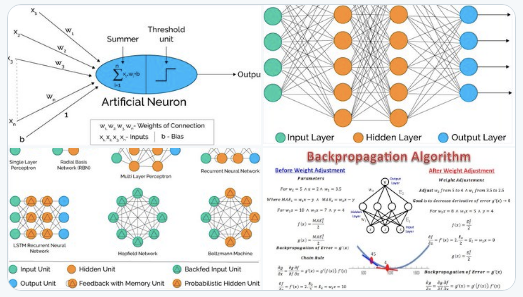Search
• Capri Granville

# Artificial Neural Networks in a Nutshell

According to Wikipedia, an ANN is based on a collection of connected units or nodes called artificial neurons, which loosely model the neurons in a biological brain. Each connection, like the synapses in a biological brain, can transmit a signal to other neurons.

An artificial neuron that receives a signal then processes it and can signal neurons connected to it.

In ANN implementations, the "signal" at a connection is a real number, and the output of each neuron is computed by some non-linear function of the sum of its inputs. The connections are called edges. Neurons and edges typically have a weight that adjusts as learning proceeds. The weight increases or decreases the strength of the signal at a connection.

Neurons may have a threshold such that a signal is sent only if the aggregate signal crosses that threshold. Typically, neurons are aggregated into layers. Different layers may perform different transformations on their inputs. Signals travel from the first layer (the input layer), to the last layer (the output layer), possibly after traversing the layers multiple times.Two interesting articles describe ANN in more details:

Artificial Neural Networks Explained

• Perceptron

• Multi-layer ANN

It also discusses several activation functions:

• Sigmoid

• Tan-h (alternative to the logistic sigmoid)

• Softmax

• ReLU

• Leaky ReLU

The activation function of a node defines the output of that node given an input or set of inputs. Finally, this article discusses the concept of backward and forward propagation. You can access this article here.